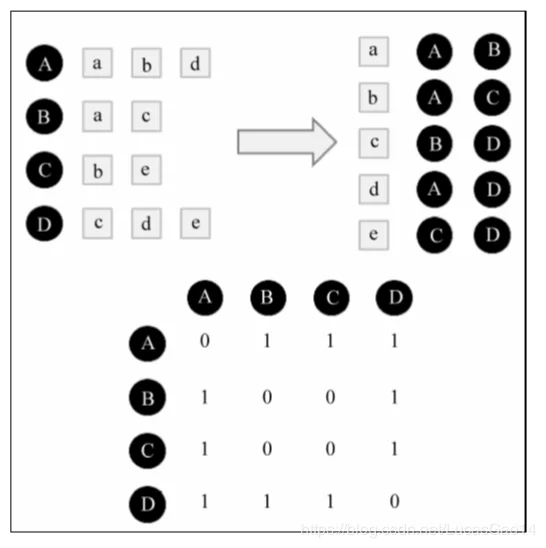# 基于用户的协同过滤系统

### 协同过滤算法的两个步骤

1. 找到和目标用户兴趣相似的用户集合
2. 找到这个集合中，用户喜欢的，且目标用户没有听说过的物品推荐给目标用户

### 如何计算用户之间的相似度/如何找到兴趣相似的用户

1. 对任何两两用户进行余弦相似度的计算
对于用户的每一个进行过行为（浏览或观看）转化为一个向量成为 aList
对于另外一个用户进行过的行为我们转化为 bList
item用户A用户B
item111
item210
item300
item411
item501
item611
item711
item800
item901

// 利用DistanceContext接口来实现距离的计算
DistanceContext distanceContext = new DistanceContext(new Cosine(aList,bList));

public class Cosine implements DistanceStrategy {
/**
* 夹角余弦
* @param aList
* @param bList
* @return
*/
@Override
public double getDistance(ArrayList<Double> aList, ArrayList<Double> bList) {
double m_ab = 0 ;           //XY的乘积和
double m_a = 0;             //X的平方和
double m_b = 0;             //Y的平方和
for (int i=0;i<aList.size();i++)
{
m_ab+=aList.get(i)*bList.get(i);
m_a+=Math.pow(aList.get(i),2);
m_b+=Math.pow(bList.get(i),2);
}
return (m_ab)/(Math.sqrt(m_a)*Math.sqrt(m_b));
}
}


Jaccard公式同样也可以计算

public class JaccardDistance implements DistanceStrategy {
/**
* 杰卡德距离
* 不同的维度的个数占“非全零维度”的比例
* @param aList
* @param bList
* @return
*/
@Override
public double getDistance(ArrayList<Double> aList, ArrayList<Double> bList) {
int count=0;        //不同维度的数量
int count1=0;       //全零维度的数量
for (int i=0;i<aList.size();i++)
{
if (!aList.get(i).equals(bList.get(i)))
count++;
if (aList.get(i)==0&&bList.get(i)==0)
count1++;
}
return count/(aList.size()-(double)count1);
}
}

1. 先对用户进行筛选，再进行相似度的计算
因为很多用户其实没有对同样的物品产生行为，我们不能把时间浪费在计算这种无谓的相似度上面。我们因此要首先计算彼此之间有对同一个物品产生了行为的用户，就是有可能相似的用户
为此我们可以先建立物品到用户的倒排表##### 总结一下以上过程就是
1. 建立倒排表
2. 建立稀疏矩阵C
3. 建立相似矩阵W
    计算相似度矩阵
for (int i=0;i<userItemList.size();i++)
for (int j = 0; j < userItemList.get(i).size(); j++)
//判断倒排表中有没有，且用户对物品进行过行为
if (!(inverted.get(j).contains(i)) && (userItemList.get(i).get(j)) != 0) {
inverted.get(j).add(i);
for (Integer integer : inverted.get(j)) {
if (integer == i)   //如果是其本身就不计算
continue;
Integer Uindex = integer;
if (!intersection.get(Uindex).contains(i) && (similarityMatrix.get(Uindex).get(i).similarity == 0)) {  //如果交集中没有i且i不是那个用户
intersection.get(Uindex).add(i);
double distance = distanceContext.getDistance(userItemList.get(Uindex), userItemList.get(i));   //计算相似度
similarityMatrix.get(i).set(Uindex, new Entry(Uindex, distance));
similarityMatrix.get(Uindex).set(i, new Entry(i, distance));
}
}
}


inverted是倒排表
intersection为交集

### 对于用户相似度计算的改进

1/(log(1+abs(N(i))))

##### 进行推荐for (int i=0;i<itemNum;i++)
{
if (userItemList.get(userIndex).get(i)!=0)          //如果本身就看过就跳过
continue;
double interest=0;
for (int j=0;j<k;j++)
{
Entry entry = array.get(j);
interest+= entry.similarity * userItemList.get(entry.user_index).get(i);        //计算兴趣
}
list.put(i,interest);
}


entry是用户相似度，里面包含了similarity和user_index
interest是用户的感兴趣程度
itemNum是物品数量

### 评论

###### Your browser is out-of-date!

Update your browser to view this website correctly. Update my browser now

×